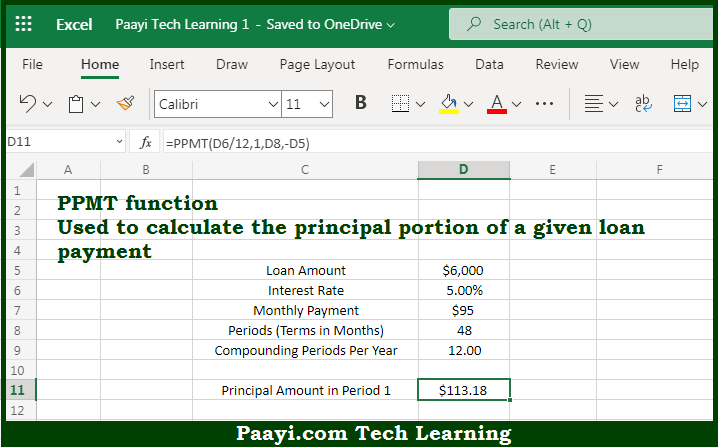# Learn How to Use Microsoft Excel PPMT Function

Written by | 0 Comments | 618 Views

In this article, you will learn how to use the Microsoft Excel PPMT function and its prime function in Microsoft Excel. You will also get to know the Microsoft Excel PPMT function return value and syntax with the help of some examples.

Microsoft Excel PPMT Function

The main purpose of the Microsoft Excel PPMT function is to get the principal payment in the given period. That implies, with the help of the PPMT function you can able to calculate the principal portion of a given loan payment. This can be understood as, PPMT can be used to get the principal amount of payment for the first period, the last period, or any period in between. So, with the help of the PPMT function, you can able to get the principal payment in the given period.

Return Value of PPMT Function

The return value will be the principal payment.

Syntax of PPMT Function

=PPMT(rate, per, nper, pv, [fv], [type])

Where the arguments:

• rate - This is the interest rate per period.
• per: This is the payment period of the interest.
• nper: This is the total number of payments for the loan.
• pv: This is the present value or total value of all loan repayments now.
• fv: This is the future value or a cash balance you required after the last payment is made and Default is 0 (optional).
• type: In case the payments are due, then 0 = end of the period and 1 = beginning of the period. Default is 0 (optional).

How to Use Microsoft Excel PPMT Function?So we know that Microsoft Excel PPMT function you can able to get the principal payment in the given period. That implies, with the help of the PPMT function you can able to calculate the principal portion of a given loan payment. This can be understood as, PPMT can be used to get the principal amount of payment for the first period, the last period, or any period in between. So, with the help of the PPMT function, you can able to get the principal payment in the given period.